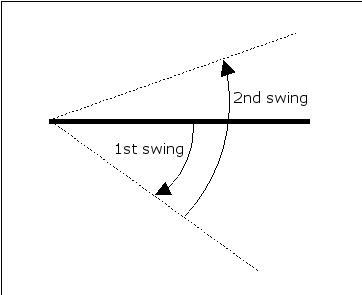### Problem Statement

We've all seen hinged doors before, perhaps in the entrance to a kitchen. Have a look at the figure below to make things clear. This particular hinged door works as follows: From "rest position" (the solid line below), the door is pushed at one end, and it swings out through an angle (in the image, this corresponds to "1st swing"). Then, when the door is released, it swings to the other side (this is shown in the image as "2nd swing"), but this time, the angle through which it swings is reduced to a known fraction of the previous angle. Then, the direction is reversed again and once more, the angle reduced by the same fraction. Once the angle drops to 5.0 degrees or below, the door doesn't swing any more, but rather, it returns to "rest position".Create a class HingedDoor which contains a method numSwings. The method takes an int initialAngle and an int reduction as input and returns an int corresponding to the number of times the door will swing before coming to rest when initially displaced by initialAngle. Remember that each time the door will swing through an angle reduction times less than the angle it swung through the previous time.

### Definition

 Class: HingedDoor Method: numSwings Parameters: int, int Returns: int Method signature: int numSwings(int initialAngle, int reduction) (be sure your method is public)

### Constraints

-initialAngle will be between 0 and 90, inclusive.
-reduction will be between 2 and 10, inclusive.

### Examples

0)

 `50` `2`
`Returns: 4`
 In this example, the door begins with an initial angle of 50 degrees. Then, the door will swing through a reduced angle of (1/2)*(50) = 25 degrees on the first swing. At this point, the door will reverse direction, and swing through an angle of (1/2)*(25) = 12.5 degrees. Continuing in this way, the door will swing once more through (1/2)*(12.5) = 6.25 degrees, and then through (1/2)*(6.25) = 3.125 degrees. At this point, the door will come to rest. Therefore, the correct return value is 4, since the door took 4 swings before coming to rest.
1)

 `45` `6`
`Returns: 2`
2)

 `23` `3`
`Returns: 2`
3)

 `3` `3`
`Returns: 0`
 Careful! The initial angle is already below 5 degrees, so the door won't swing at all, but rather, return to rest position immediately.
4)

 `73` `5`
`Returns: 2`

#### Problem url:

http://www.topcoder.com/stat?c=problem_statement&pm=3531

#### Problem stats url:

http://www.topcoder.com/tc?module=ProblemDetail&rd=8072&pm=3531

NeverMore

#### Testers:

PabloGilberto , brett1479 , Olexiy

#### Problem categories:

Simple Math, Simulation